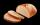Jam cakes

Mom baked a third of plum jam cakes, one third cheesecakes and 18 poppy. How many cakes she had bake?

Result

x =  54

Solution:1/3 x = 18

x = 54

x = 54

Calculated by our simple equation calculator.

Leave us a comment of example and its solution (i.e. if it is still somewhat unclear...):Be the first to comment!To solve this example are needed these knowledge from mathematics:

Need help calculate sum, simplify or multiply fractions? Try our fraction calculator. Do you have a linear equation or system of equations and looking for its solution? Or do you have quadratic equation?

Next similar examples:

1. CakesOn the bowl were a few cakes. Jane ate one-third of them, Dana ate a quarter of those cakes that remained. a) What part (of the original number of cakes) Dana ate? b) At least how many cakes could be (initially) on thebowl?
2. Cakes1/3 poppy cake, 1/3 apple, 15 pieces of cheese. How many are totally cakes?
3. Discount saleAfter the discount, the computer costs 9600, - CZK. How much did it cost when the price was reduced: a) by half b) by a third c) by one fifth and then by 160 CZKMom bake cookies. Rolo took 2/9 of all cookies, Michal 3/9. How many cookies ate Rolo if Michal had 9.
5. Forest nurseryIn the forest nursery after winter, they found that 1/10 stems died out of them. For them, they land 193 new spruces. How many spruces are in the forest nursery?Several pieces of bread were in the store. Half of them plus a half of bread bought a cookbook. Of the remaining bread, half of it plus half of the bread was bought by Mr. Novák. The last bread was bought by Ms. Small. How many breads were in the store at.
7. Lengths of the poolMiguel swam 6 lengths of the pool. Mat swam 3 times as far as Miguel. Lionel swam 1/3 as far as Miguel. How many lengths did mat swim?
8. If-then equationIf 5x - 17 = -x + 7, then x =
9. Eq1Solve equation: 4(a-3)=3(2a-5)
10. Simple equation 5Solve equation with fractions: X × 3/8 = 1/2
11. Equation 20In given equation: 8/9-4/5=2/9+x, find x
12. Fraction and a decimalWrite as a fraction and a decimal. One and two plus three and five hundredths
13. Fraction to decimalWrite the fraction 3/22 as a decimal.Added together and write as decimal number: LXVII + MLXIVZdeněk picked up 15 l of water from a 100-liter full-water barrel. Write a fraction of what part of Zdeněk's water he picked.An ant climbs 2/5 of the pole on the first hour and climbs 1/4 of the pole on the next hour. What part of the pole does the ant climb in two hours?Write the mixed number as an improper fraction. 166 2/3